## Finding the Volume of a Cone

### Learning Outcomes

• Find the volume of cones

The first image that many of us have when we hear the word ‘cone’ is an ice cream cone. There are many other applications of cones (but most are not as tasty as ice cream cones). In this section, we will see how to find the volume of a cone.

In geometry, a cone is a solid figure with one circular base and a vertex. The height of a cone is the distance between its base and the vertex.  The cones that we will look at in this section will always have the height perpendicular to the base. See the image below.

The height of a cone is the distance between its base and the vertex.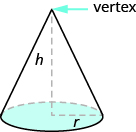Earlier in this section, we saw that the volume of a cylinder is $V=\pi {r}^{2}h$. We can think of a cone as part of a cylinder. The image below shows a cone placed inside a cylinder with the same height and same base. If we compare the volume of the cone and the cylinder, we can see that the volume of the cone is less than that of the cylinder.

The volume of a cone is less than the volume of a cylinder with the same base and height.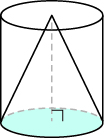In fact, the volume of a cone is exactly one-third of the volume of a cylinder with the same base and height. The volume of a cone is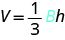Since the base of a cone is a circle, we can substitute the formula of area of a circle, $\pi {r}^{2}$, for $B$ to get the formula for volume of a cone.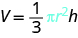In this course, we will only find the volume of a cone, and not its surface area.

### Volume of a Cone

For a cone with radius $r$ and height $h$ .### example

Find the volume of a cone with height $6$ inches and radius of its base $2$ inches.

Solution

 Step 1. Read the problem. Draw the figure and label it with the given information.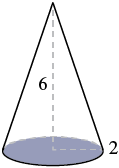Step 2. Identify what you are looking for. The volume of the cone Step 3. Name. Choose a variable to represent it. Let $V$ = volume. Step 4. Translate. Write the appropriate formula. Substitute. (Use $3.14$ for $\pi$ ) $V=\Large\frac{1}{3}\normalsize\pi {r}^{2}h$ $V\approx \Large\frac{1}{3}\normalsize 3.14{\left(2in\right)}^{2}\left(6in\right)$ Step 5. Solve. $V\approx 25.12in^3$ Step 6. Check: We leave it to you to check your calculations. Step 7. Answer the question. The volume is approximately $25.12$ cubic inches.

### example

Marty’s favorite gastro pub serves french fries in a paper wrap shaped like a cone. What is the volume of a conic wrap that is $8$ inches tall and $5$ inches in diameter? Round the answer to the nearest hundredth.

### try it

In the following video we provide another example of how to find the volume of a cone.

## Contribute!

Did you have an idea for improving this content? We’d love your input.